Visitors Online: 0 | Saturday 14th December 2019CBSE Guess > Papers > Important Questions > Class XII > 2008 > Maths > Maths By Mrs. Geethanjali Bhatt CBSE CLASS XII
Q. 1.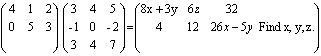Ans. x = 1, z = 4, y = 3 Q. 2.Ans. -1 Q. 3. Using the method of matrices solve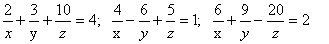Ans.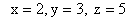Q. 4. Using properties of determinants solve for x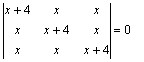Ans.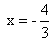Q. 5. If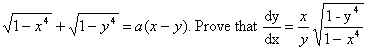Q. 6. If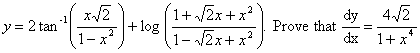Q. 7.Ans. a = 1, b = -1 Q. 8.Ans. a = 1/2 Q. 9. Solve for x : 2tan-1 (cosx) = tan-1 (2cosx) Ans. x = 2n P ± P /2 Q. 10. (a) If tan-1 x + tan-1 y + tan-1 z = P /2 .Evaluate xy + yz + xz Ans. 1 (b) If tan-1 x + tan-1 y + tan-1 z = P Evaluate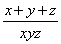Q. 11. Show that sin.cot -1 tan cos -1 x = x sec2 (tan-1 2)+cosec2 (cot-13) = 15 Q. 12. Prove that the function f : R -> {x E R -1 [0, 1] be defined by f(x) =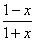g : [0, 1] --> [0, 1] be defined by g(x) = 4x (1 - x) find fog and gof Ans.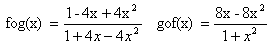Q. 21. Differentiate logAns.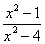Q. 22. y =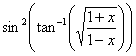Ans. 1/2